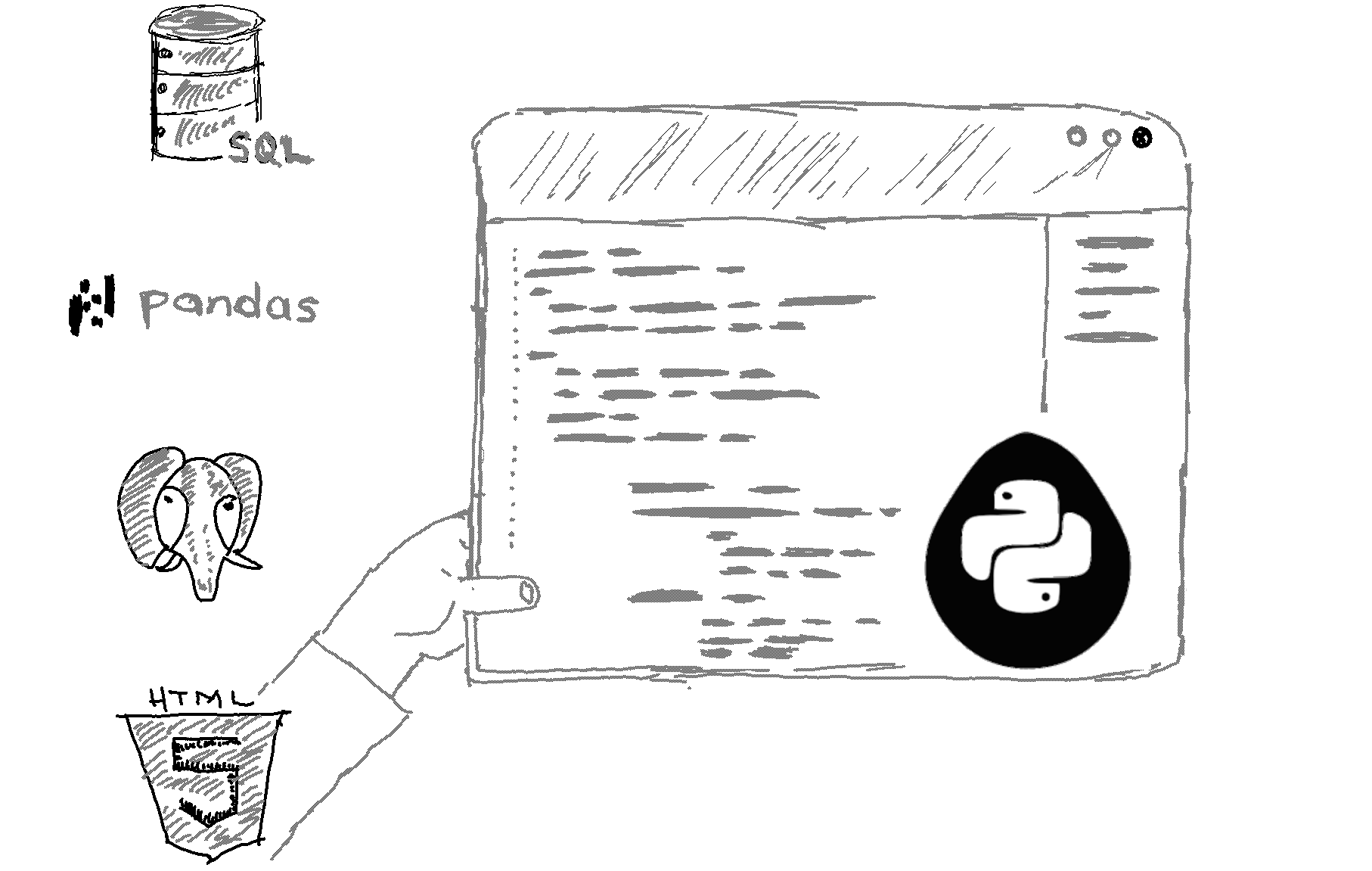# Here is how to llimit floats to two decimal points in Python.

```x = 3.14159265

# Round x to two decimal points
y = round(x, 2)

print(y) ```

Output
`3.14`

Explanation

To limit a float to two decimal points in Python, you can use the round function. The round function takes a number and the number of decimal points to round to as arguments, and returns the rounded number. In this case, the float x is rounded to two decimal points, resulting in the value 3.14.

Alternatively, you can use the format function to format a float as a string with a fixed number of decimal points. Here is an example of how to use format to limit a float to two decimal points:

```x = 3.14159265

# Format x as a string with two decimal points
y = "{:.2f}".format(x)

print(y)  # Output: "3.14"```
The format function takes a format string as the first argument and the value to format as the second argument. The format string specifies the desired formatting for the value, and the {:.2f} syntax specifies that the value should be formatted as a float with two decimal points.

Both round and format can be used to limit floats to a fixed number of decimal points in Python. You can choose the one that best fits your needs.

Related HowTos
##### Recommended Course

Learn Flask development and learn to build cool apps with our premium Python course on Udemy.